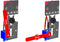Unlock your students potential with these 2 free Lesson Plans for our ACCESS:bit for the BBC microbit. Students will learn how to code the micro:bit to control an access barrier with these classroom-ready teaching resources.They have been designed to introduce Key Stage 3 students to embedded systems. Both lessons include curriculum mapping and also practical challenges for KS3 students. We've gone into as much detail as possible so you can get these into the classroom with as little fuss as possible.

## Teachers Lesson Plans For ACCESS:bit For microbit:

Firstly, the ZIP file for these lesson plans contains two PDFs, one for each lesson. There is a brief overview of each lesson plan below, for more details please refer to the lesson plans themselves.This is an introductory lesson for Key Stage 3 students to embedded systems. The lesson involves the teacher discussing how we make access barriers to coordinate traffic, first in plain English, then through algorithms, then through code. The teacher will write the algorithms on the board and then the students will create the code using the algorithm as a guide. The recommended ratio of students to ACCESS:bits is 2:1.This is the second lesson for Key Stage 3 students to embedded systems. The lesson involves the teacher discussing how we trigger access barriers to move, first in plain English, then through algorithms, then through code. The teacher will write the algorithms on the board and then the students will create the code using the algorithm as a guide. The recommended ratio of students to ACCESS:bits is 2:1.